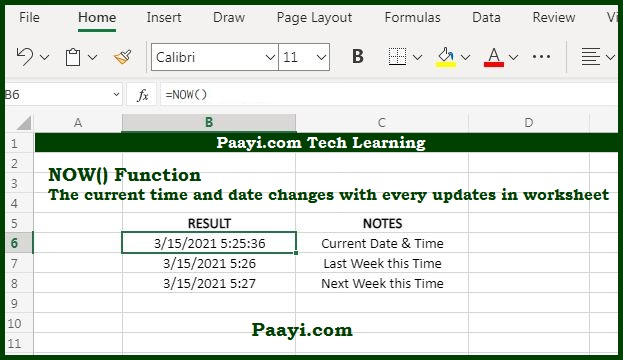# Learn How to Use Microsoft Excel NOW Function

Written by | 0 Comments | 710 Views

In this article, you will learn how to use the Microsoft Excel NOW function and its prime function in Microsoft Excel. You will also get to know the Microsoft Excel NOW function return value and syntax with the help of some examples.

## Microsoft Excel NOW Function

The purpose of the Microsoft Excel NOW function is to get the current time and date. That means using the NOW function; you can return the current date and time, which is updated continuously when you open or change the worksheet. You can't use any arguments with the NOW function; you can format the value returned by the NOW function as a date or as a date with time applying a number format.

### NOW Function Return Value

The return value will be the serial number representing the date and time as per the Excel format.

= NOW( )

## How to Use Microsoft Excel NOW Function?As we know, how we can return the current date and time with the NOW function. In the NOW function, you can't use any arguments but requires an empty parenthesis.

The value returned using the NOW function will be continuously updated when the worksheet is refreshed, or each time the value is entered or updated. You can use the F9 to force the worksheet to recalculate and update the value.